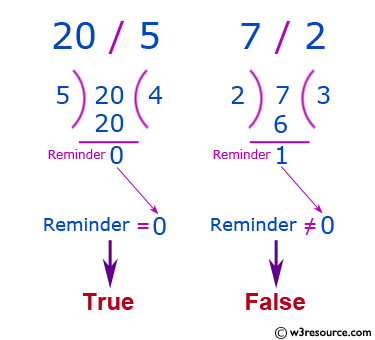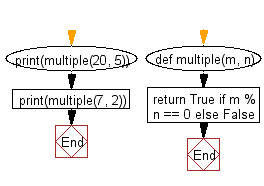﻿ Python: Function to check whether a number is divisible by another number - w3resource# Python: Function to check whether a number is divisible by another number

## Python Basic: Exercise-147 with Solution

Write a Python function to check whether a number is divisible by another number. Accept two integers values form the user.

Sample Solution :-

Python Code :

``````def multiple(m, n):
return True if m % n == 0 else False

print(multiple(20, 5))
print(multiple(7, 2))
``````

Sample Output:

```True
False
```

Pictorial Presentation:Flowchart:## Visualize Python code execution:

The following tool visualize what the computer is doing step-by-step as it executes the said program:

Python Code Editor :

Have another way to solve this solution? Contribute your code (and comments) through Disqus.

What is the difficulty level of this exercise?

Test your Python skills with w3resource's quiz

﻿

## Python: Tips of the Day

Unpack variables from iterable:

```# One can unpack all iterables (tuples, list etc)
>>> a, b, c = 1, 2, 3
>>> a, b, c
(1, 2, 3)

>>> a, b, c = [1, 2, 3]
>>> a, b, c
(1, 2, 3)
```